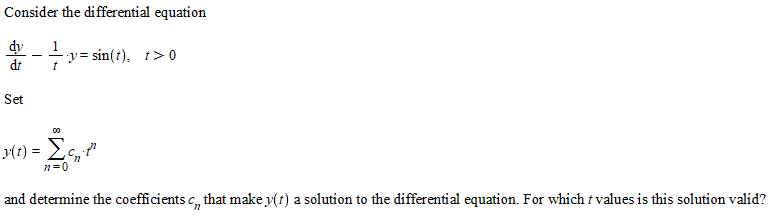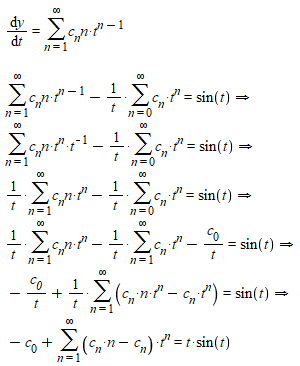# 1st Order Differential Equation - Power Series Method

## Homework Statement## The Attempt at a SolutionI have deliberately made several obvious steps, because I keep ending up here. However I have no idea what to do from here. I thought about the fact, that differential equations have the solution ##x = x_{HOM} + x_{Inhom}##, but the ##x_{HOM}## ends up the same, except equal 0, which suggests that the only solution is ##c_0 = -t \cdot sin(t)##. as all ##c_n=0, n \geq 1, t \in (0,\infty)##. But that can't be right, because that actually constitute a solution?